# 1 2 Measuring and Constructing Segments Measuring Segments

• Slides: 111. 2 Measuring and Constructing Segments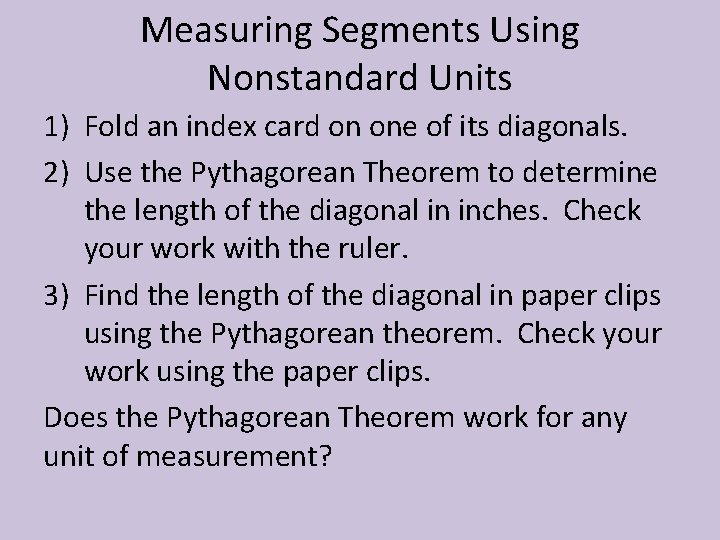Measuring Segments Using Nonstandard Units 1) Fold an index card on one of its diagonals. 2) Use the Pythagorean Theorem to determine the length of the diagonal in inches. Check your work with the ruler. 3) Find the length of the diagonal in paper clips using the Pythagorean theorem. Check your work using the paper clips. Does the Pythagorean Theorem work for any unit of measurement?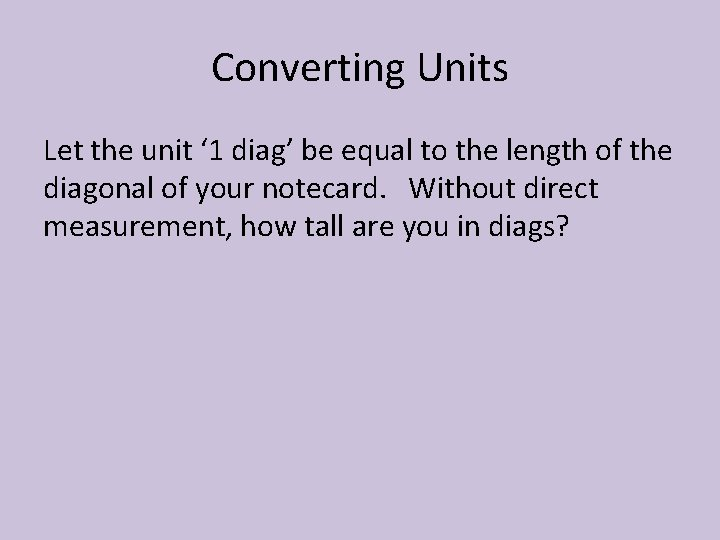Converting Units Let the unit ‘ 1 diag’ be equal to the length of the diagonal of your notecard. Without direct measurement, how tall are you in diags?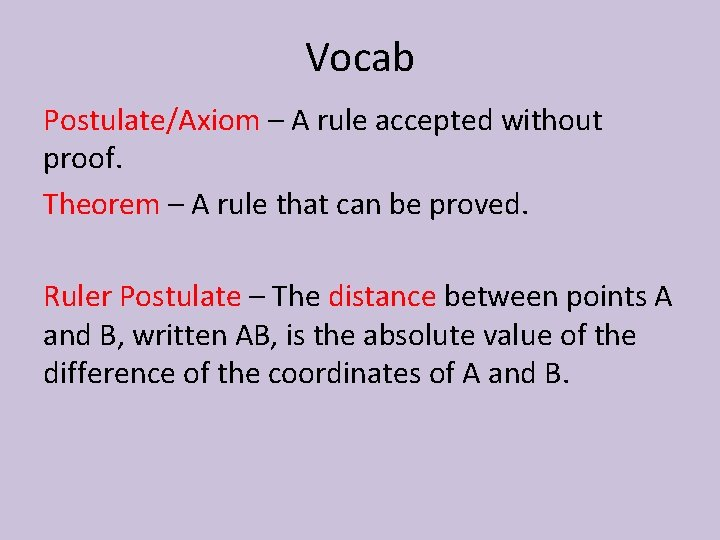Vocab Postulate/Axiom – A rule accepted without proof. Theorem – A rule that can be proved. Ruler Postulate – The distance between points A and B, written AB, is the absolute value of the difference of the coordinates of A and B.1) What is the length of the segment above? 2) Come up with two separate equations that describe the length of PR if P has a coordinate of 7, R has a coordinate of x and the length is 10. Bonus: What are two possible values for x?Construction – a geometric drawing created using tools (like a protractor or compass). Congruent Segments – line segments that have the same length. Investigation: Draw a line segment using a straight edge called AB. How can you use the compass to make a segment CD such that AB = CD.Lengths are equal. Segments are congruent.When three points are collinear, you can say that one point is between the other two. Segment Addition Postulate – If B is between A and C, then AB + BC = AC.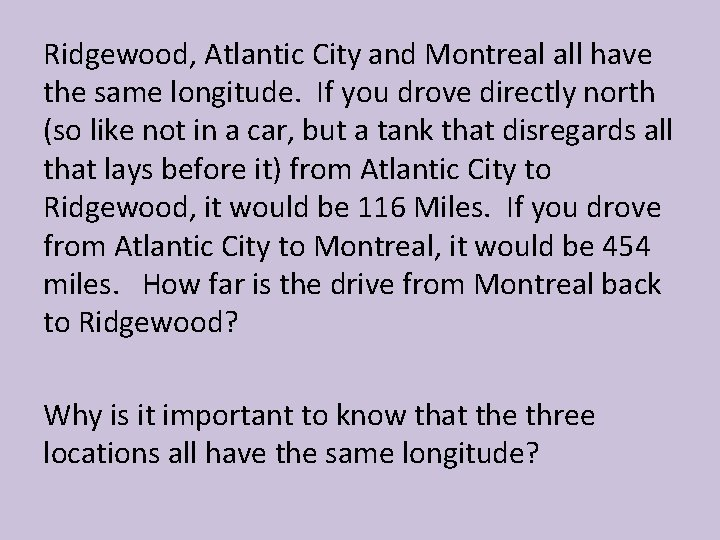Ridgewood, Atlantic City and Montreal all have the same longitude. If you drove directly north (so like not in a car, but a tank that disregards all that lays before it) from Atlantic City to Ridgewood, it would be 116 Miles. If you drove from Atlantic City to Montreal, it would be 454 miles. How far is the drive from Montreal back to Ridgewood? Why is it important to know that the three locations all have the same longitude?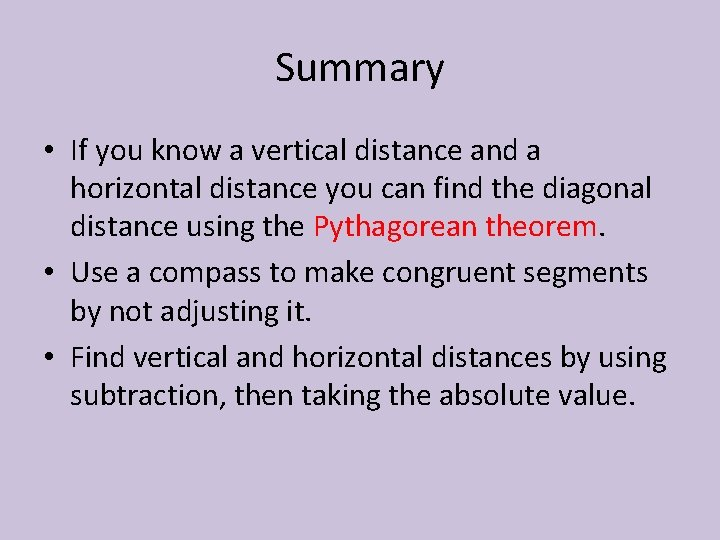Summary • If you know a vertical distance and a horizontal distance you can find the diagonal distance using the Pythagorean theorem. • Use a compass to make congruent segments by not adjusting it. • Find vertical and horizontal distances by using subtraction, then taking the absolute value.NOTECARD GAME